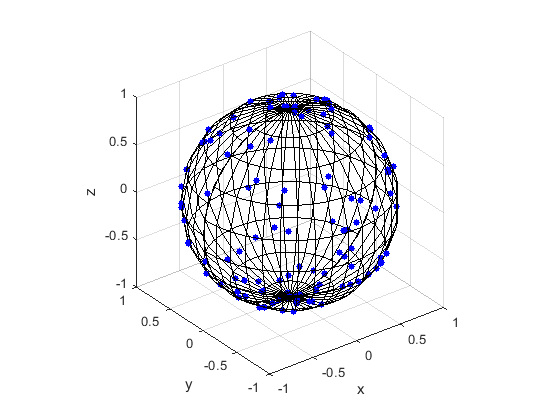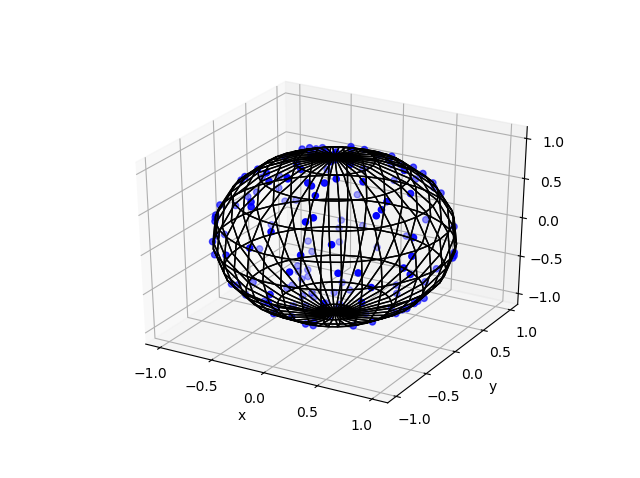## Simulating a Poisson point process on a sphere

In this post I’ll describe how to simulate or sample a homogeneous Poisson point process on the surface of a sphere. I have already simulated this point process on a rectangle, triangle disk, and circle.

Of course, by sphere, I mean the everyday object that is the surface of a three-dimensional ball, where this two-dimensional object is often denoted by $$S^2$$.  Mathematically, this is a generalization from a Poisson point process on a circle, which is slightly simpler than randomly positioning points on a disk.  I recommend reading those two posts first, as a lot of the material presented here builds off them.

I have not needed such a simulation in my own work, but I imagine there are many reasons why you would want to simulate a Poisson point process on a sphere. As some motivation, we can imagine these points on a sphere representing, say, meteorites or lightning hitting the Earth.

The generating the number of points is not difficult. The trick is positioning the points on the sphere in a uniform way.  As is often the case, there are various ways to do this, and I recommend this post, which lists the main ones.  I will use two methods that I consider the most natural and intuitive ones, namely using spherical coordinates and normal random variables, which is what I did in the post on the circle.

Incidentally, a simple modification allows you to scatter the points uniformly inside the sphere, but you would typically say ball in mathematics, giving a Poisson point process inside a ball; see below for details.

## Steps

As always, simulating a Poisson point process requires two steps.

##### Number of points

The number of points of a Poisson point process on the surface of a sphere of radius $$r>0$$ is a Poisson random variable with mean $$\lambda S_2$$, where $$S_2=4\pi r^2$$ is the surface area of the sphere. (In this post I give some details for simulating or sampling Poisson random variables or, more accurately, variates.)

### Locations of points

For any homogeneous Poisson point process, we need to position the points uniformly on the underlying space, which is in this case is the sphere. I will outline two different methods for positioning the points randomly and uniformly on a sphere.

##### Method 1: Spherical coordinates

The first method is based on spherical coordinates $$(\rho, \theta,\phi)$$, where the radial coordinate $$\rho\geq 0$$, and the angular coordinates $$0 \leq \theta\leq 2\pi$$ and $$0\leq \phi \leq \pi$$. The change of coordinates gives $$x=\rho\sin(\theta)\cos(\phi)$$, $$y=\rho\sin(\theta)\sin(\phi)$$, and $$z=\rho\cos(\phi)$$.

Now we use Proposition 1.1 in this paper. For each point, we generate two uniform variables $$V$$ and $$\Theta$$ on the respective intervals $$(-1,1)$$ and $$(0,2\pi)$$. Then we place the point with the Cartesian coordinates

$$X = r \sqrt{1-V^2} \cos\Theta,$$

$$Y = r \sqrt{1-V^2}\sin\Theta,$$

$$Z=r V.$$

This method places a uniform point on a sphere with a radius $$r$$.

###### How does it work?

I’ll skip the precise details, but give some interpretation of this method. The random variable $$\Phi := \arccos V$$ is the $$\phi$$-coordinate of the uniform point, which implies $$\sin \Phi=\sqrt{1-V^2}$$, due to basic trigonometric identities.  The area element in polar coordinates is $$dA = \rho^2 \sin\phi d\phi d\theta$$, which is constant with respect to $$\theta$$. After integrating with respect to $$\phi$$,  we see that the random variable $$V=\cos\Phi$$ needs to be uniform (instead of $$\Phi$$) to ensure the point is uniformly located on the surface.

##### Method 2: Normal random variables

For each point, we generate three standard normal or Gaussian random variables, say, $$W_x$$, $$W_y$$, and $$W_z$$, which are independent of each other. (The term standard here means the normal random variables have mean $$\mu =0$$ and standard deviation $$\sigma=1$$.)  The three random variables are the Cartesian components of the random point. We rescale the components by the Euclidean norm, then multiply by the radius $$r$$, giving

$$X=\frac{rW_x}{(W_x^2+W_y^2+W_z^2)^{1/2}},$$

$$Y=\frac{rW_y}{(W_x^2+W_y^2+W_z^2)^{1/2}},$$

$$Z=\frac{rW_z}{(W_x^2+W_y^2+W_z^2)^{1/2}}.$$

These are the Cartesian coordinates of a point uniformly scattered on a  sphere with radius $$r$$ and a centre at the origin.

###### How does it work?

The procedure is somewhat like the Box-Muller transform in reverse. In the post on the circle setting,  I gave an outline of the proof, which I recommend reading. The joint density of the normal random variables is from a multivariate normal distribution with zero correlation. This joint density is constant on the sphere, implying that the angle of the point $$(W_x, W_y, W_z)$$ will be uniformly distributed.

The vector formed from the normal variables $$(W_x, W_y,W_z)$$ is a random variable with a chi distribution.  We can see that the vector from the origin to the point $$(X,Y,Z)$$ has length one, because we rescaled it with the Euclidean norm.

## Plotting

Depending on your plotting software, the points may more resemble points on an ellipsoid than a sphere due to the different scaling of the x, y and z axes. To fix this in MATLAB, run the command: axis square. In Python, it’s not straightforward to do this, as it seems to lack an automatic function, so I have skipped it.

## Results

I have presented some results produced by code written in MATLAB and Python. The blue points are the Poisson points on the sphere. I have used a surface plot (with clear faces) to illustrate the underling sphere in black.

##### MATLAB##### Python

Note: The aspect ratio in 3-D Python plots tends to squash the sphere slightly, but it is a sphere.## Code

The code for all my posts is located online here. For this post, the code in MATLAB and Python is here.  In Python I used the library mpl_toolkits for doing 3-D plots.

## Poisson point process inside the sphere

Perhaps you want to simulate a Poisson point process inside the ball.  There are different ways we can do this, but I will describe just one way, which builds off Method 1 for positioning the points uniformly. (In a later post, I will modify Method 2, giving a way to uniformly position points inside the ball.)

For this simulation method, you need to make two simple modifications to the simulation procedure.

##### Number of points

The number of points of a Poisson point process inside a sphere of radius $$r>0$$ is a Poisson random variable with mean $$\lambda V_3$$, where $$V_3=4\pi r^3$$ is the volume of the sphere.

### Locations of points

We will modify Method 1 as outlined above. To sample the points uniformly in the sphere, you need to generate uniform variables on the unit interval $$(0,1)$$, take their cubic roots, and then, multiply them by the radius $$r$$. (This is akin to the step of taking the square root in the disk setting.) The random variables for the angular coordinates are generated as before.